##### The Japanese Maple specialist
Direct order Contact Help / Services Newsletter Pay in 3 times without charges, from 135 euros in your shopping cart (France residents only)# Shelf to displaySearch

Japanese MaplesBonsai soil and fertiliserAcer seeds and more Young maples and bonsaiHostasWind bells and chimesSaxifragesVarieties introduced into Europe

Packing and shipping charges

# Acer palmatum set of 50 trees

Japanese maple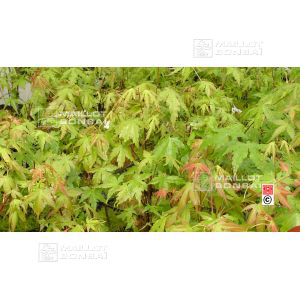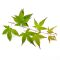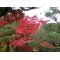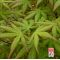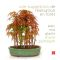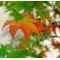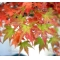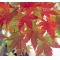ref. : 10449

Product unavailable

###### Description

Delivered in plastic pots.

Height 20/30 cm.

Pack of 50 acer palmatum for planting in forest bonsai style. The trees have pink-green foliage in spring, green in summer with pinky brown young shoots, followed by a yellow-orange-red canopy in autumn. The plants are descended from seedlings and may vary slightly in leaf shape and colour.

#palmatum 3.8 #bonsai 3.6 #trees 3.5 #young 3.5 #acer 3.4 #maples 2.6 #green 2.5 #delivered 1.9 #descended 1.9 #seedlings 1.9

Formule
(( ROUND((CHAR_LENGTH(b.article_nom)-CHAR_LENGTH(REPLACE(b.article_nom, 'palmatum', '')))/LENGTH('palmatum')) + ROUND((CHAR_LENGTH(b.article_description)-CHAR_LENGTH(REPLACE(b.article_description, 'palmatum', '')))/LENGTH('palmatum')) ) * 3.8) + (( ROUND((CHAR_LENGTH(b.article_nom)-CHAR_LENGTH(REPLACE(b.article_nom, 'trees', '')))/LENGTH('trees')) + ROUND((CHAR_LENGTH(b.article_description)-CHAR_LENGTH(REPLACE(b.article_description, 'trees', '')))/LENGTH('trees')) ) * 3.5) + (( ROUND((CHAR_LENGTH(b.article_nom)-CHAR_LENGTH(REPLACE(b.article_nom, 'acer', '')))/LENGTH('acer')) + ROUND((CHAR_LENGTH(b.article_description)-CHAR_LENGTH(REPLACE(b.article_description, 'acer', '')))/LENGTH('acer')) ) * 3.4) + (( ROUND((CHAR_LENGTH(b.article_nom)-CHAR_LENGTH(REPLACE(b.article_nom, 'green', '')))/LENGTH('green')) + ROUND((CHAR_LENGTH(b.article_description)-CHAR_LENGTH(REPLACE(b.article_description, 'green', '')))/LENGTH('green')) ) * 2.5) + (( ROUND((CHAR_LENGTH(b.article_nom)-CHAR_LENGTH(REPLACE(b.article_nom, 'seedlings', '')))/LENGTH('seedlings')) + ROUND((CHAR_LENGTH(b.article_description)-CHAR_LENGTH(REPLACE(b.article_description, 'seedlings', '')))/LENGTH('seedlings')) ) * 1.9) + (( ROUND((CHAR_LENGTH(b.article_nom)-CHAR_LENGTH(REPLACE(b.article_nom, 'delivered', '')))/LENGTH('delivered')) + ROUND((CHAR_LENGTH(b.article_description)-CHAR_LENGTH(REPLACE(b.article_description, 'delivered', '')))/LENGTH('delivered')) ) * 1.9) + (( ROUND((CHAR_LENGTH(b.article_nom)-CHAR_LENGTH(REPLACE(b.article_nom, 'descended', '')))/LENGTH('descended')) + ROUND((CHAR_LENGTH(b.article_description)-CHAR_LENGTH(REPLACE(b.article_description, 'descended', '')))/LENGTH('descended')) ) * 1.9) + (( ROUND((CHAR_LENGTH(b.article_nom)-CHAR_LENGTH(REPLACE(b.article_nom, 'slightly', '')))/LENGTH('slightly')) + ROUND((CHAR_LENGTH(b.article_description)-CHAR_LENGTH(REPLACE(b.article_description, 'slightly', '')))/LENGTH('slightly')) ) * 1.8) + (( ROUND((CHAR_LENGTH(b.article_nom)-CHAR_LENGTH(REPLACE(b.article_nom, 'followed', '')))/LENGTH('followed')) + ROUND((CHAR_LENGTH(b.article_description)-CHAR_LENGTH(REPLACE(b.article_description, 'followed', '')))/LENGTH('followed')) ) * 1.8) + (( ROUND((CHAR_LENGTH(b.article_nom)-CHAR_LENGTH(REPLACE(b.article_nom, 'planting', '')))/LENGTH('planting')) + ROUND((CHAR_LENGTH(b.article_description)-CHAR_LENGTH(REPLACE(b.article_description, 'planting', '')))/LENGTH('planting')) ) * 1.8)

## Secure payment## Delivery

Our logistic partners :